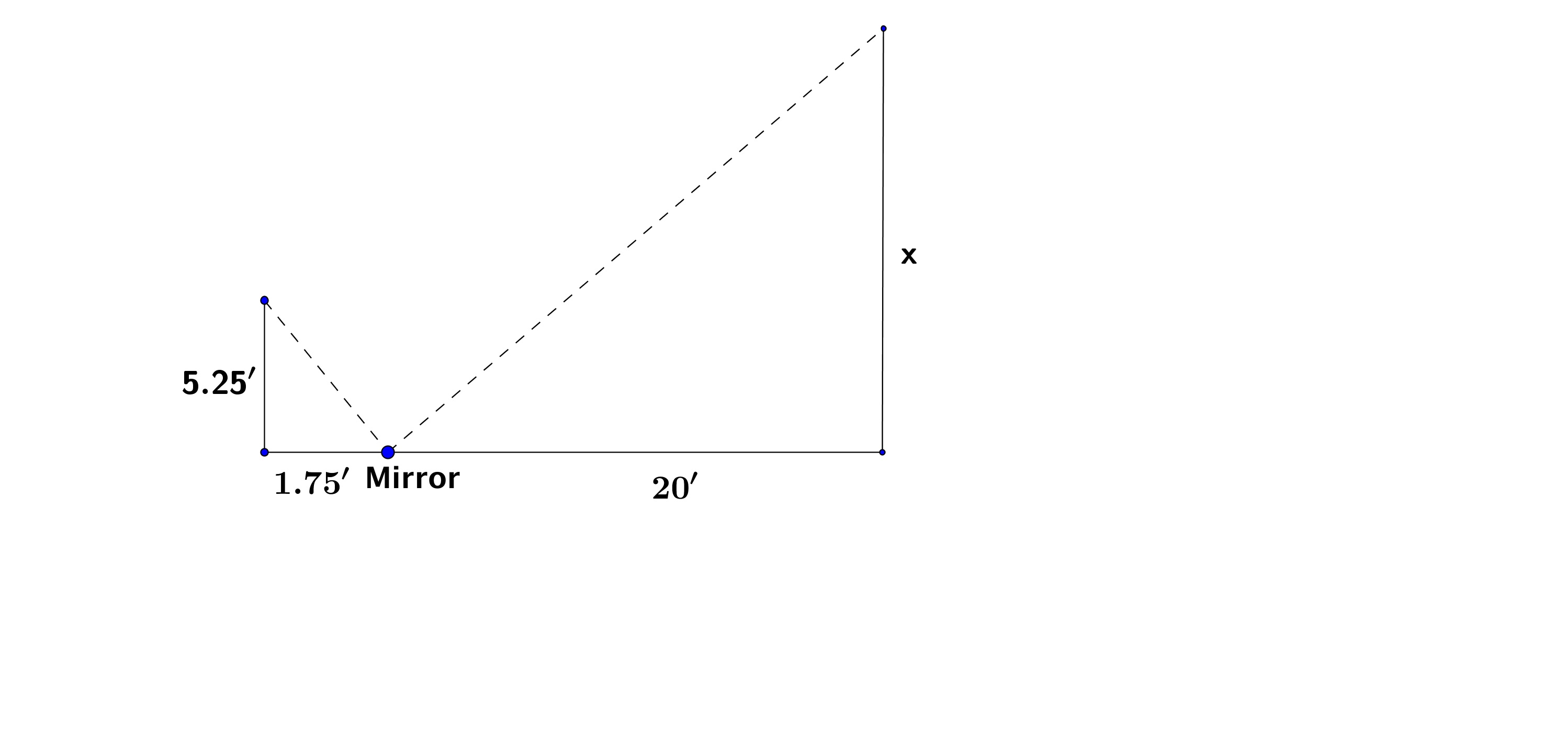# GEOM 3 | Lesson 4 | Practice (Solutions)

1.  $$\dfrac{5}{20} = \dfrac{5.5}{x}$$;  The building is $$22’$$ tall.2.  $$\dfrac{1.75}{20} = \dfrac{5.25}{x}$$.  The library is $$60’$$ tall.3.  $$\dfrac{3}{15} = \dfrac{6.25}{x}$$;  The flagpole is  $$31.25’$$ high.4.  $$\dfrac{3.5}{8} = \dfrac{12}{x+12}$$;  You stand $$15.4’$$ from the net.5.   $$\dfrac{2}{9} = \dfrac{6.25}{x}$$ 0r tree is  $$28.125’$$ tall.